(Called 'Manipulate Variables' in iNZight Lite
Convert variables to new types, create new variables, and rename existing ones)

iNZight assumes that data sets are in rows = cases by columns = variables format. For example, the cases (also often called units) may be individual people and the columns = variables contain different types of "measures" on those people.

By default, if all the values of a variable are numbers, then that variable will be treated as as a numeric variable.

If any of the values contains even one alphabetic character, then (by default) the whole variable will be treated as a categorical variable (i.e., one that gives group membership). The one exception is the value `NA`, which is treated as a missing-value code in both numeric and categorical variables.

## Page Contents: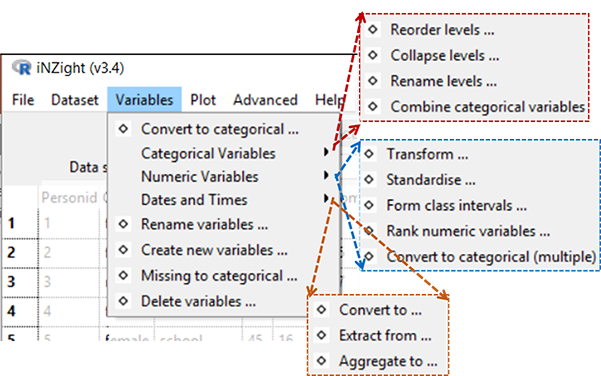## Convert to Categorical

Creates a categorical version of a numeric variable (for more sophisticated version see ...Convert to Categorical (Multiple) ...).

## Categorical Variables

### Reorder Levels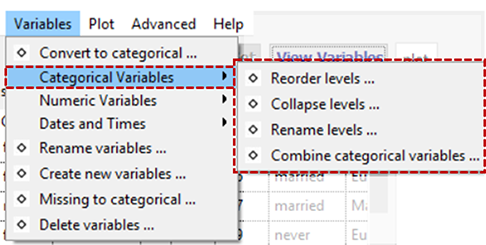By default, the levels of a categorical variable are displayed in alpha-numeric order. This enables you to change from the default order of display to something more natural; e.g. from {"adolescent", "adult", "child", "elder"} to {"child", "adolescent", "adult", "elder"}.

### Collapse Levels

Combine levels within a categorical parent variable to make a new variable with a smaller number of levels. (The levels of a categorical variable are the set of unique, or distinct, values it takes).

### Rename Levels

Change the names of the levels of a categorical variable, e.g. from (ages) {"< 12", "12-17", "18-69", "> 69"} to {"child", "adolescent", "adult", "elder"}.

### Combine Categorical Variables

Take two categorical variables and create a new categorical variable whose levels are all combinations of those two (e.g., the combinations of ethnicity and gender).

## Numeric Variables

### Transform Variables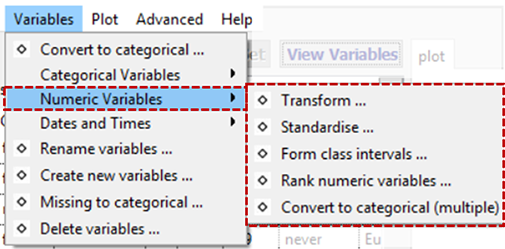Creates a new variable that is a transformed version of the parent variable. Transformations available are `log` (base `e` or base 10), exponential, square, square root and reciprocal.

### Standardise Variables

Create a standardised version of a variable (or z-score) by subtracting the mean of the variable from each value and dividing by its standard deviation.

### Form Class Intervals

Create a categorical variable whose levels are class intervals of a numeric variable. For example, take `age` and create `age.f` with levels {"{0,20]", "(20,60]", "(60,110]"}.

### Rank Numerical Variables

Creates a new numeric variable containing the rank, or order of the selected variable. This is often used when the order of the values (but not the values themselves) is of interest.

For example, the variable `age = {15, 12, 19, 16}` would result in the new variable `age.rank = {2, 1, 4, 3}`.

### Convert to Categorical (Multiple)

This has the same result as the previous "Convert to Categorical" tool, however it allows users to convert multiple variables at once.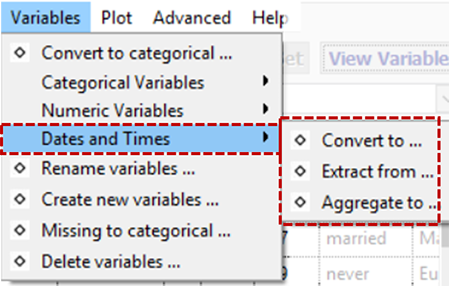## Dates and Times

### (Date-times) Convert to ...

Convert a variable to a standard dates and times (POSIXct) format and saves as a new variable.

In the example that follows, Time.stamp is a categorical variable that we wish to convert to a variable that iNZight recognises as a datetime variable.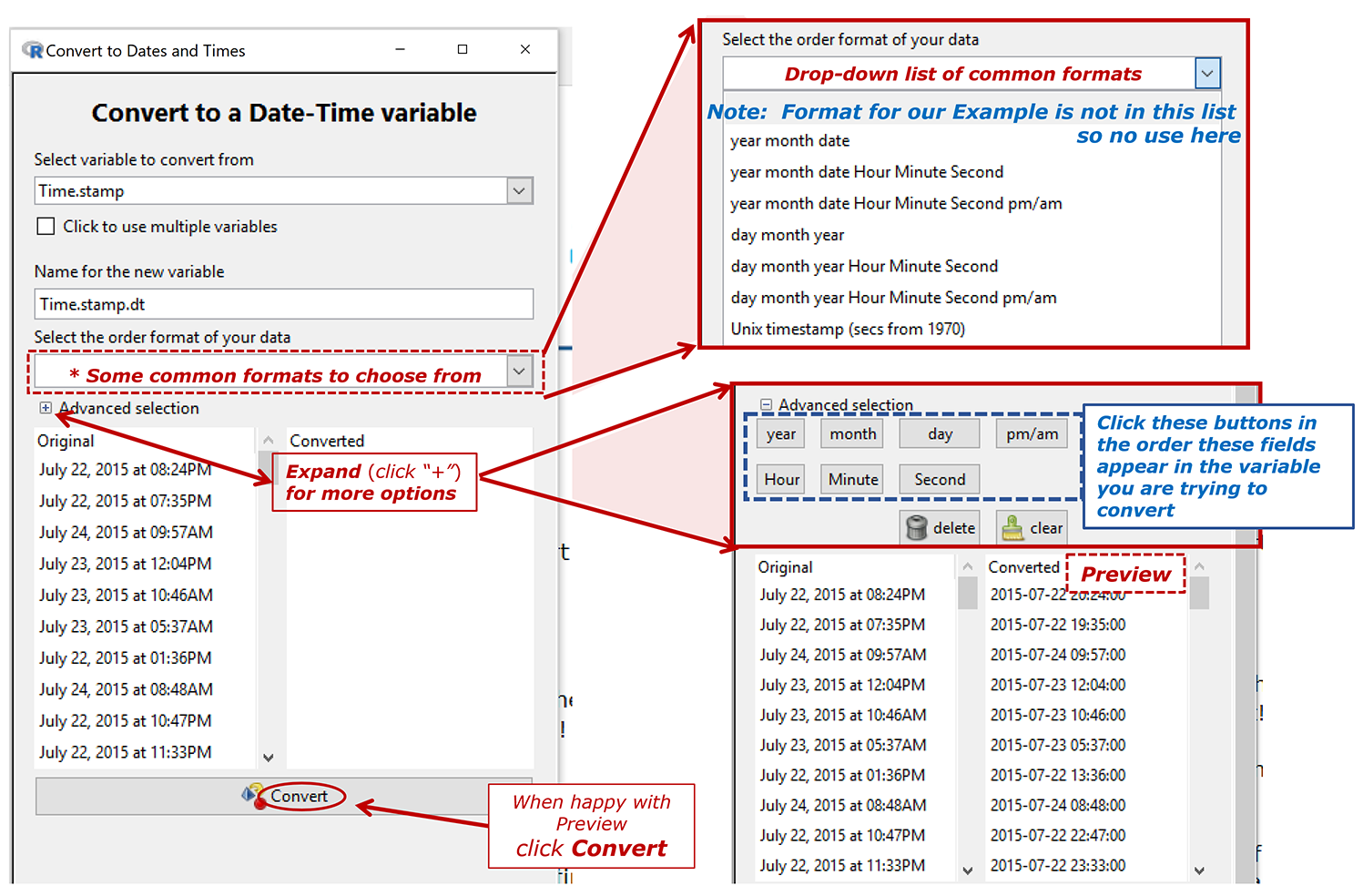### (Date-times) Extract from ...

Creates a new variable by extracting specific component (e.g. data, year, time...) from a dates and times variable in a large variety of levels of detail and of format.

• The pattern is to expand the option (click the "+") referring to the most detailed level of information you want in the result. The preview will show you what the result of your choice will look like.

In the example that follows, Time.stamp.dt is a categorical variable that we wish to convert to a variable that iNZight recognises as a datetime variable Timestamp.dt. What we want to do is extract a new variable containing the Year and the decimal part of the Year ("Decimal Year")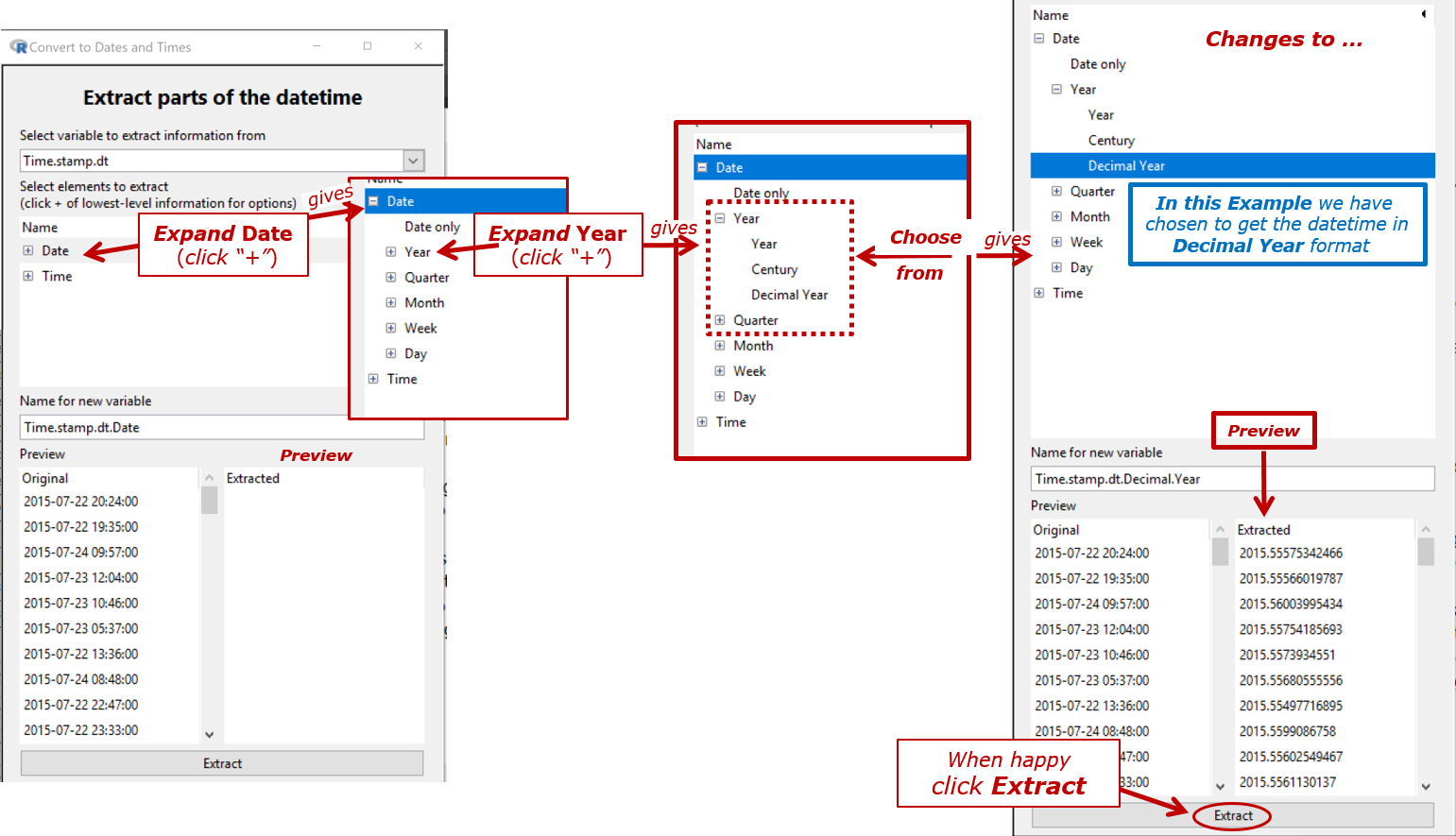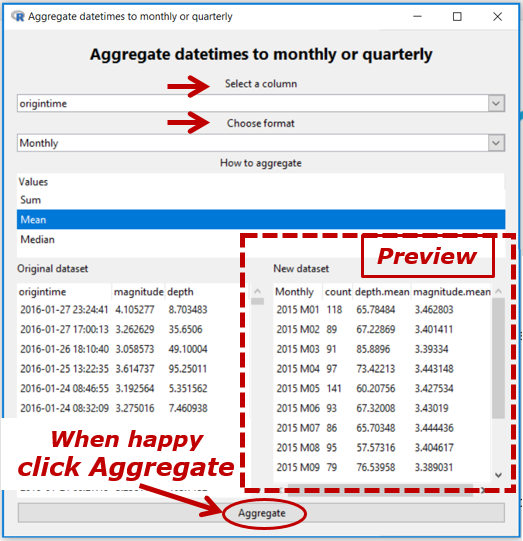### (Date-times) Aggregate over ...

Converts to

• a weekly (e.g. 2019 W7), monthly (e.g. 2019 M5), quarterly (e.g. 2019 Q3) or yearly (e.g. 2019) variable in the form that the time series variable likes

and aggregates using sum, mean, or median.

In the Example, `origintime` is a date-time variable [thus, annotated `(t)` in View Variables] and we have chosen to convert it to monthly (`yyyy Myy`) with all of the values in the same month aggregated to their `mean`.

The `count` column shows how many values have been aggregated. If there are missing values present in a variable being aggregated, then another column of the form `variable.missing`. Missing values are ignored when aggregating.

In the Example below a categorical variable in monthly (`yyyy Mzz`) format is aggregated to quarterly (`yyyy Qz`).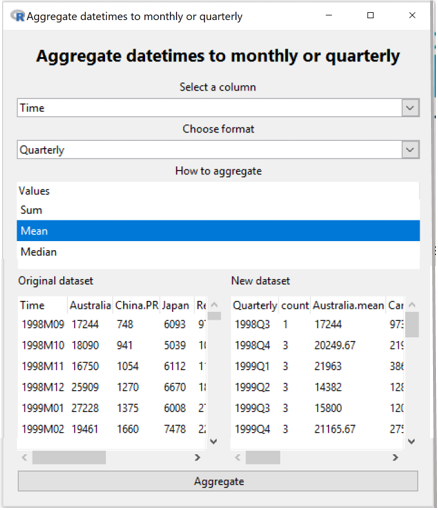### Rename Variables

Rename variables in the dataset. Especially useful for variables created by iNZight.

### Create New Variable

A very flexible facility for creating new variables from existing variables, essentially by doing arithmetic on them.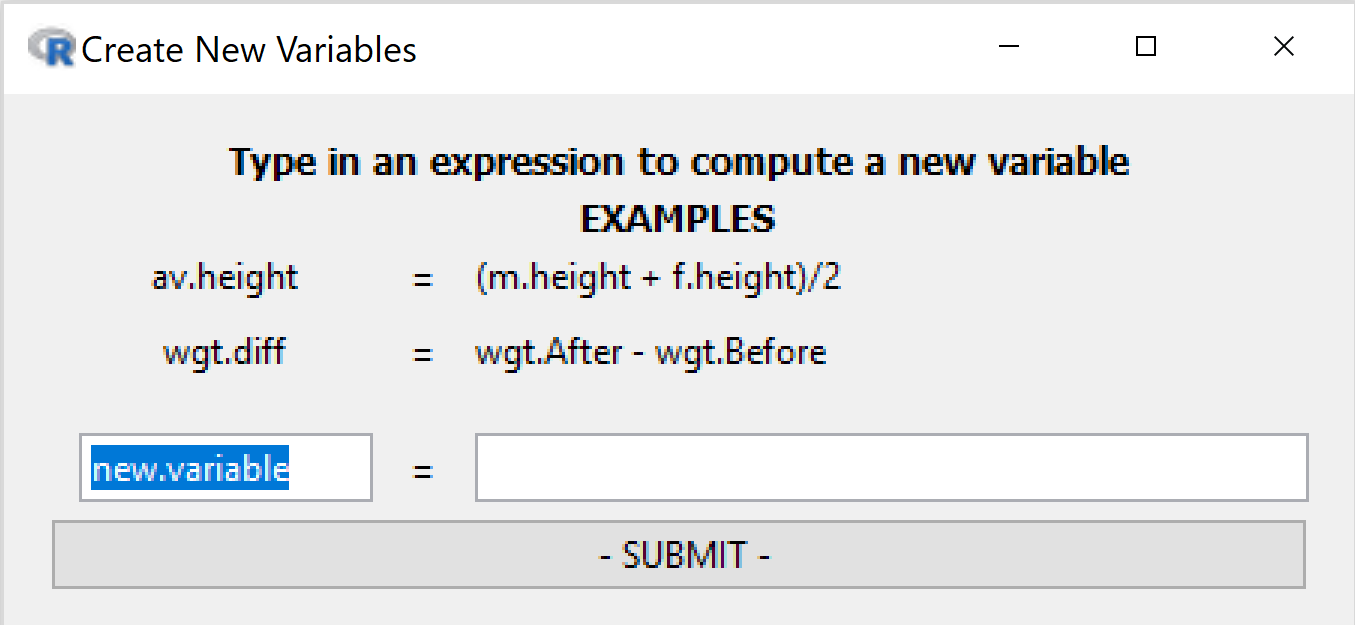Left-hand box: desired name for new variable
Right-hand box: any valid R expression

Examples

• `income = hours * payrate`
• `weight.diff = end.weight - begin.weight`
• `average.weight = ( begin.weight + endweight) / 2`

## Missing to Categorical

• Categorical variables: Any missing observations for the variable we be given a new level, `missing`. All others remain the same.

• Numeric variables: The variable will be converted into a categorical variable with two levels: `missing` and `observed`.

## Delete Variables

Delete variables from the dataset. Click variable names using the Shift and Control keys to choose multiple variables for deletion.